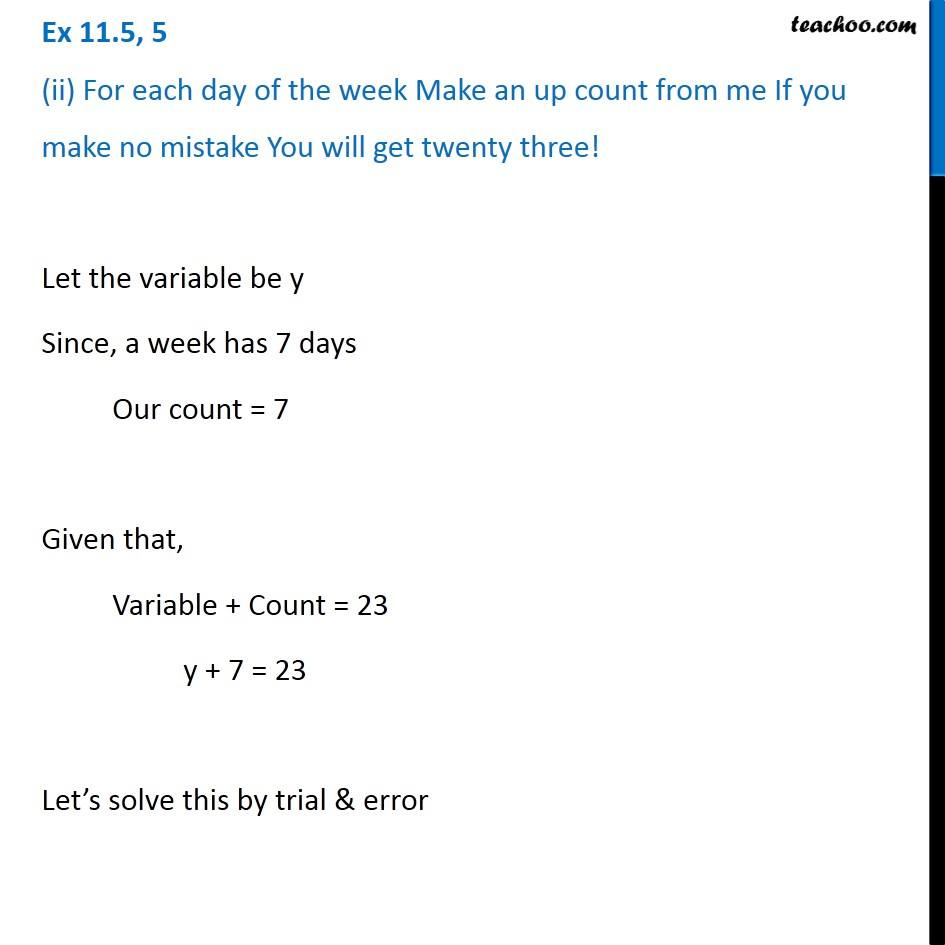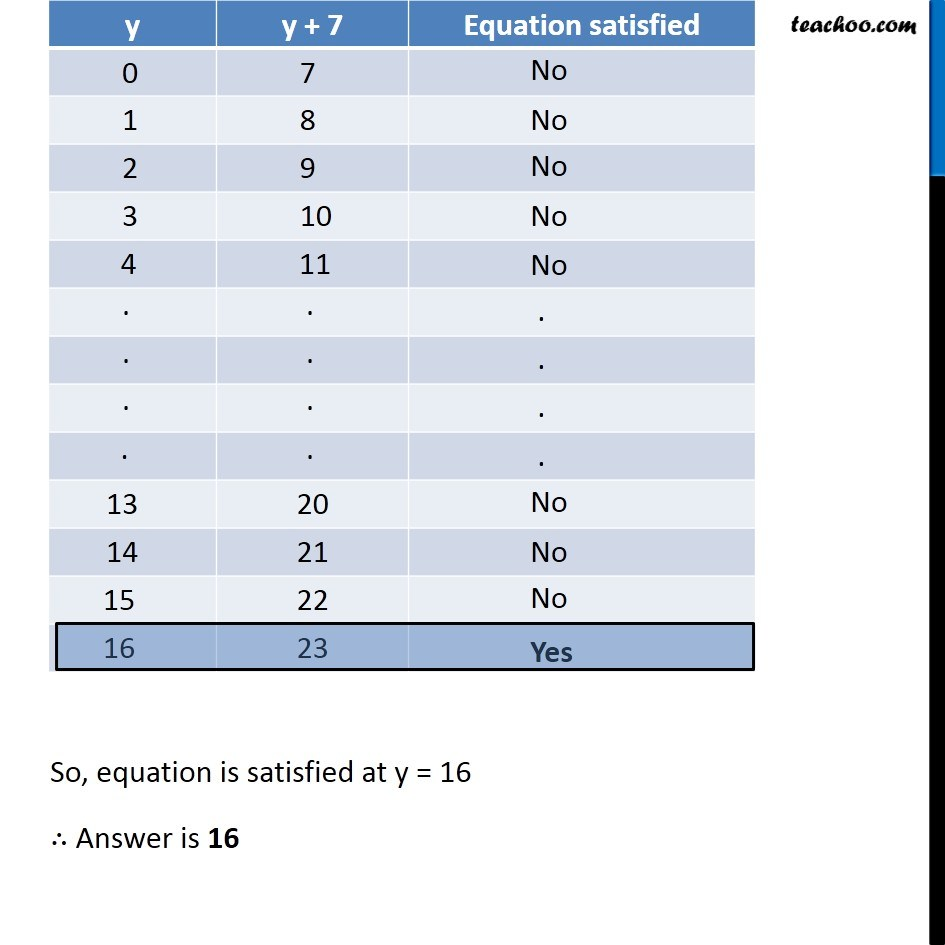1. Chapter 11 Class 6 Algebra
2. Serial order wise
3. Ex 11.5

Transcript

Ex 11.5, 5 (ii) For each day of the week make an up count from me if you make no mistake you will get twenty three! Let the variable be y Since, a week has 7 days Our count = 7 Given that, Variable + Count = 23 y + 7 = 23 Let’s solve this by trial & error So, equation is satisfied at y = 16 ∴ Answer is 16